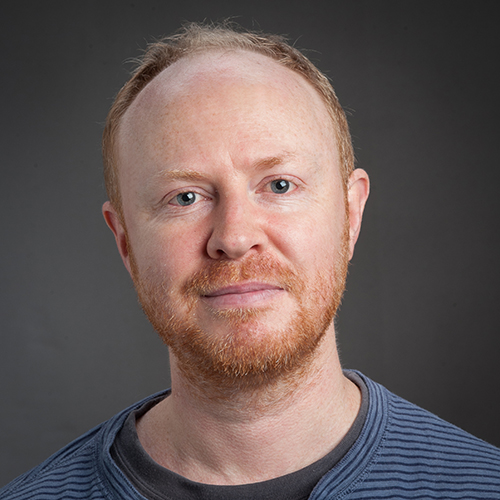# Logic and algebra

Research in the Logic and Algebra Group builds on ideas from combinatorics, mathematical logic, and general algebra.

The School of Mathematics and Statistics has significant research strengths in two main areas of logic: computability theory and descriptive set theory. Computability theory begins with the fundamental concept of an algorithm that can be made precise using Turing machines. The ideas of computability theory can be applied to a wide range of topics in mathematics. For example, researchers in the School are world-leaders in understanding the computability-theoretic properties of algebra. The School's researchers were also instrumental in developing the modern theory of algorithmic randomness. This theory links ideas of compressibility and definability to produce a robust notion of when an individual object is random.

Descriptive set theory uses techniques from logic to understand definability in a wide range of settings. Recently descriptive set theorists have applied these techniques to many branches of mathematics.

In combinatorics, the School's main strength in is in matroid theory. Matroids are abstract mathematical objects that arise from geometrical configurations. The geometry of matroid theory is quite different from the classical geometry of the ancient Greek mathematicians. The configurations may exist in a version of space with four, five, or more dimensions. This type of geometry arises quite naturally when considering applications in computer science. For example, matroid theory is closely linked to coding theory—the branch of mathematics that lets us send information accurately even when errors are introduced during transmission.

We have strong links with the Philosophy programme and the School of Engineering and Computer Science, and we hold joint seminars involving staff and postgraduate students frequently.

## Researchers

### Prof Rod Downey

Emeritus Professor

Research Fellow

School of Mathematics and Statistics|School of Mathematics and Statistics

### Prof Noam Greenberg

Professor of Mathematics
School of Mathematics and Statistics

Professor Noam Greenberg is interested in computability theory, reverse mathematics, algorithmic randomness and applications of computability to analysis, computable algebra, extensions of computability to higher domains, and the interactions between computability and set theory.

### Prof Geoff Whittle

Research Fellow

School of Mathematics and Statistics|School of Mathematics and Statistics### Dillon Mayhew

Associate Professor Dillon Mayhew works in matroid theory, and is especially interested in using tools from logic and the theory of computation to study matroid questions. In particular, he is investigating formal logical languages that we can use to make statements about matroids. Matroids can be defined in these languages, and so can many natural properties of matroid. But not every matroid property can be expressed in this way: for example, Dillon Mayhew, Geoff Whittle, and their colleague Mike Newman have shown that the class of matroids arising from vector spaces cannot be characterised using the natural logical language for matroids known as monadic second-order logic. The study of logical languages also gives insight into the difficulty, or otherwise, of testing matroid properties computationally. For example, a matroid property that can be stated in monadic second-order logic can often be efficiently tested.

### Dr Dan Turetsky

Senior Lecturer in Mathematics
School of Mathematics and Statistics

## Current projects

### Structure theory for matroids representable over infinite fields (Prof Geoff Whittle)

Matroids are, in essence, finite geometrical configurations. A natural way to obtain a matroid is from an algebraic structure known as a field. Historically, the focus has been on matroids obtained from finite fields. But it is also natural to consider matroids obtained from infinite fields such as the familiar field of real numbers used in coordinate geometry. The primary goal of this project is to resolve a twenty-year old conjecture of Robertson and Seymour with the long-term vision of using the solution to gain insight into the structure of matroids that can be obtained from infinite fields.

### Uncountable structures and effective properties (Prof Noam Greenberg)

Model theory, set theory, and computability theory are areas of mathematical logic that have diverged over the years. Nonetheless, basic core ideas are common to all three, above all, the treatment of language as a mathematical object that can be studied formally and used to gain important insights about algebra, computable processes, and the foundations of mathematics. This project aims to develop new connections between these areas. We will use a generalisation of computability to uncountable domains to study algebraic objects such as groups, and general classification of mathematical structures. We examine standard Turing computability in contrast with its generalisations; this allows us to separate the incidental from the fundamental in classical results.

Approximation techniques common to both set theory and computability allow us to make connections between regularity properties of the real numbers on the one hand, and the computational power of these numbers on the other. For example, identifying patterns in all algorithmically defined numbers is closely related to the number of statistical tests (null sets) required to capture all real numbers. We aim to identify the rules that govern these connections and apply these to solve open problems.

### The Mathematics of Computation (Prof Rod Downey)

Computation is ubiquitous in modern society, yet the mathematics of computation lags way behind both the applications of computation and the development of classical mathematics. This project contributes to our understanding of the computable content of mathematics. The kinds of basic questions we expect to solve include: When can computational tasks be performed? How hard are they? How does theory align with practice? Can classical results be proven using computational methods? These general questions are underpinned by technical longstanding open problems as well as general research programs in completely new spheres of investigation.